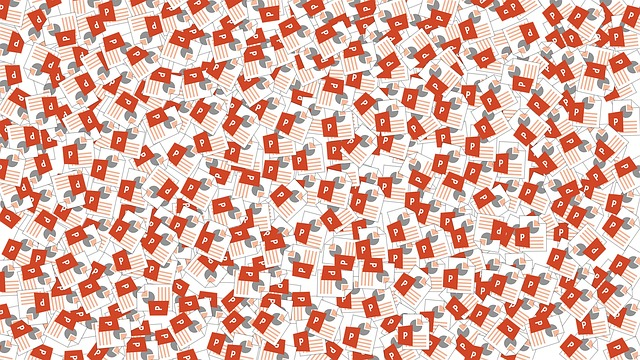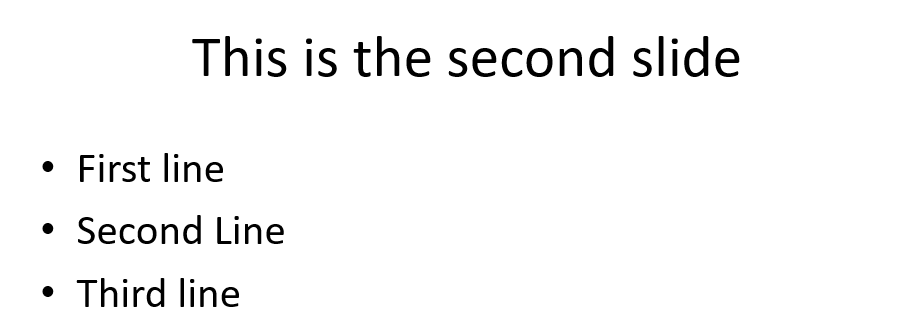Want to share your content on R-bloggers? click here if you have a blog, or here if you don't.In my last post, we discussed how to create and read Word files with R’s officer package. This article will expand on officer by showing how we can use it to create PowerPoint reports.

## Getting started

```library(officer)

```

Next, we’ll create a PowerPoint object in R using the read_pptx function.

```pres <- read_pptx()

```

To add a slide, we use the add_slide function. The first slide we’ll create is the title slide. We specify the type of slide in the layout parameter. There’s several other possibilities here including “Title and Content”, “Blank”, “Title Only”, “Comparison”, “Two Content”, and “Section Header”.

Secondly, we use ph_with to add the title text.

```# add title slide
pres <- add_slide(pres, layout = "Title Only", master = "Office Theme")

pres <- ph_with(pres, value = "My first presentation", location = ph_location_type(type = "title"))

```

Next, let’s add another slide. This time we’ll have a title and content.

```pres <- add_slide(pres, layout = "Title and Content", master = "Office Theme")
pres <- ph_with(pres, value = "This is the second slide", location = ph_location_type(type = "title"))
pres <- ph_with(pres, value = c("First line", "Second Line", "Third line"), location = ph_location_type(type = "body"))

```Now, what if we want to add a table? Let’s create a sample data frame and add it to a new slide. Again, we’ll use ph_with to add the content (data frame in this case) to the new slide. This time we just need to set the value parameter equal to our data frame object.

```# create sample data frame
frame <- data.frame(a = 1:10, b = 11:20, c = 21:30)

# create slide to hold table
pres <- add_slide(pres, layout = "Title and Content", master = "Office Theme")
pres <- ph_with(pres, value = "Table Example", location = ph_location_type(type = "title"))

# add data frame to PowerPoint slide
pres <- ph_with(pres, value = frame, location = ph_location_type(type = "body"))

```Plots and images can also be added to the PowerPoint document. In the code below we add a ggplot object to a new slide.

```library(ggplot2)
pres <- add_slide(pres, layout = "Blank", master = "Office Theme")
sample_plot <- ggplot(data = frame) + geom_point(mapping = aes(1:10, a),
size = 3) + theme_minimal()

pres <- ph_with(pres, value = sample_plot, location = ph_location_fullsize())

```

External images can be loaded like this:

```pres <- add_slide(pres)
pres <- ph_with(pres, external_img("sample_image.png", width = 2, height = 3),
location = ph_location_type(type = "body"), use_loc_size = FALSE )

```

Note how in this case, we wrap the name of the image file along with the width and height sizes we want inside the external_img function.

Font sizes and colors can be adjusted using the fp_text function. In the example below we created two paragraphs – the first one is bold, while the second one is green.

```# create bold text object with size 24 font
bold <- fp_text(font.size = 24, bold = TRUE)

# create green Arial text object with size 24 font
green <- fp_text(font.size = 24, color = "green", font.family = "Arial")

# create block list of two paragraphs with the above font specifics
pars <- block_list(fpar(ftext("This line is bold", bold)), fpar(ftext("This line is green and Arial", green)))

pres <- add_slide(pres, layout = "Title and Content", master = "Office Theme") %>% ph_with(pars, location = ph_location_type(type = "body"))

``````pres <- add_slide(pres)

pres <- ph_hyperlink(pres, href = "https://theautomatic.net")

```